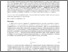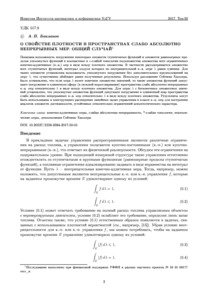# On a density property of weakly absolutely continuous measures. General case

(2017). On a density property of weakly absolutely continuous measures. General case. Izvestiya Instituta Matematiki i Informatiki 2 (50) 3-12.Preview
Text
50-01.pdf - Published Version

## Abstract

It is shown that some set of all step functions (and the set of all uniform limits of such functions) allows an embedding into a compact subset (with respect to weak-star topology) of the set of all finitely additive measures of bounded variation in the form of an everywhere dense subset. In particular, we consider the set of all step functions (the set of all uniform limits of such functions) such that an integral of absolute value of the functions with respect to nonnegative finitely additive measure λ is equal to unity. For these sets, the possibility of embedding is proved without any additional assumptions on λ; this generalizes the previous results. Using the Sobczyk-Hammer decomposition theorem, we show that for λ with the finite range, the above-mentioned sets of functions allow an embedding into the unit sphere (in the strong norm-variation) of weakly absolutely continuous measures with respect to λ in the form of an everywhere dense subset. For λ with an infinite range, the above-mentioned sets of functions allow an embedding into the unit ball of weakly absolutely continuous measures with respect to λ in the form of an everywhere dense subset. The results can be helpful for an extension of linear impulse control problems in the class of finitely additive measures to obtain robust representations of reachable sets given by constraints of asymptotic character.

Item Type: Article finitely additive measures, weak absolute continuity, weak-star topology, nonatomic or atomless measures, Sobczyk-Hammer decomposition Advanced Systems Analysis (ASA) Luke Kirwan 12 Jan 2018 09:17 27 Aug 2021 17:29 http://pure.iiasa.ac.at/15046View Item